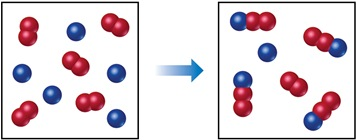# Problem: The following diagram represents a reaction shown going to completion. Each molecule in the diagram represents 0.1 mol, and the volume of the box is 1.0 L.Calculate the value of Kc at 25 oC.

###### FREE Expert Solution

We are asked to calculate the value of Kp at 25 oC.

R → Red sphere

B → Blue sphere

Given:

Reactants

• 5  molecules R2
• 5 molecules B

Products

• 4 molecules BR2
• 1  molecule R2
• 1 molecules B

The reactions is

R+ B ↔BR2

79% (165 ratings)###### Problem Details
The following diagram represents a reaction shown going to completion. Each molecule in the diagram represents 0.1 mol, and the volume of the box is 1.0 L.Calculate the value of Kc at 25 oC.

Frequently Asked Questions

What scientific concept do you need to know in order to solve this problem?

Our tutors have indicated that to solve this problem you will need to apply the Chemical Equilibrium concept. You can view video lessons to learn Chemical Equilibrium. Or if you need more Chemical Equilibrium practice, you can also practice Chemical Equilibrium practice problems.

What professor is this problem relevant for?

Based on our data, we think this problem is relevant for Professor Strathman's class at IOWA.